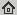# Modelling Two-Phase Flow in a Micro-Model with Local Thermal Non-Equilibrium on the Darcy Scale

International Journal of Heat and Mass Transfer, 88: 822--835, 2015
Abstract: Loosening local equilibrium assumptions in two-phase flow in porous media gives rise to new, unknown variables. More specically, when loosening the local thermal equilibrium assumption, one has to describe the heat transfer between multiple phases, present at the same mathematical point. In this paper, we calibrate a macro-scale mathematical model which is free of local equilibrium assumptions to experimental observations. We emphasize the correct determination and upscaling of necessary input parameters from the experimental data achieved by image analysis. By choosing an appropriate scaling parameter, we are able to reproduce experimental measurements satisfactorily. This is a first step towards quantifying heat transfer in two-phase flow in porous media. Ultimately, our aim is to find the limits of the applicability of local equilibrium assumptions in two-phase flow in porous media.

## BibTex reference

```@Article{Ron15a,
author       = "P. Nuske and O. Ronneberger and N. K. Karadimitriou and R. Helmig and S. M. Hassanizadeh",
title        = "Modelling Two-Phase Flow in a Micro-Model with Local Thermal Non-Equilibrium on the Darcy Scale",
journal      = "International Journal of Heat and Mass Transfer",
volume       = "88",
pages        = "822--835",
year         = "2015",
url          = "http://lmb.informatik.uni-freiburg.de/Publications/2015/Ron15a"
}
```